# Class 6 Maths NCERT Solutions for Chapter 14 Practical Geometry Ex – 14.6

## Practical Geometry

Question 1.
Draw ZPOQ of measure 75° and find its line of symmetry.

Solution:
Steps of Construction: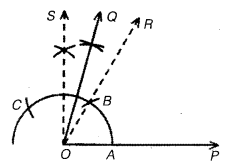1. Draw a ray OP.
2. Draw ∠POR = 60° and ∠POS = 90°.
3. Draw OQ, the bisector of ∠ROS.
Then,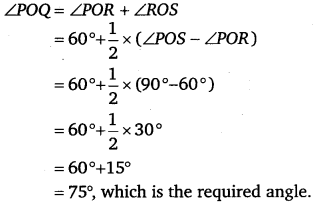Question 2.
Draw an angle of measure 147° and construct its bisector.

Solution:
Steps of Construction:

1. Draw a ray OA.
2. Place the protractor on OA such that its centre falls on the initial point O and 0 -180 line lies along OA.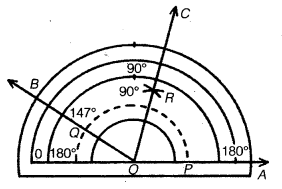3. Mark a point B on the paper against the mark of 147° on the protractor.
4. Remove the protractor and draw OB. Then, the ∠AOB so obtained is the required angle such that ZAOB = 147°.

To construct its bisector:

1. With centre O and a convenient radius draw an arc cutting sides OA and OB at P and Q respectively.
2. With centre P and radius >$\frac { 1 }{ 2 }$ PQ, draw an arc.
3. With centre Q and the same radius, as in the previous step, draw another arc intersecting the arc drawn in the previous step at R.
4. Join OR and produce it to form ray OC.
Then, the ∠AOC so obtained is the bisector of ∠AOB.

Question 3.
Draw a right angle and construct its bisector.

Solution:
Steps of Construction:

To draw an angle of 90°:

1. Draw a ray OA.
2. With O as centre and any convenient radius, draw an arc cutting at P.
3. With P as centre and same radius, draw an arc cutting the arc of step 2 at Q.
4. With Q as centre and the same radius as in steps 2 and 3, draw an arc cutting the arc drawn in step 3 at R.
5. With Q as centre and the same radius, draw an arc.
6. With R as centre and the same radius, draw an arc cutting the arc drawn in step 5 at B.

To draw its bisector:

1. With P as centre and radius >$\frac { 1 }{ 2 }$ PT, draw an arc in the interior of ∠AOC.
2. With T as centre and the same radius, an in step 1, draw another arc intersecting the arc in step 1 at D.
3. Join OD and produce it to any point E.
Then, ∠AOE so obtained is the bisector of ∠AOC.

Question 4.
Draw an angle of measure 153° and divide it into four equal parts.

Solution:
Steps of Construction: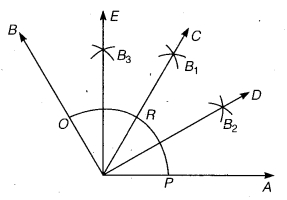1. With the help of the protractor, draw ∠AOB = 153°.
2. With centre O and any convenient radius, draw an arc cutting OA and OB at P and Q respectively.
3. With centre P and radius >$\frac { 1 }{ 2 }$ PQ, draw an arc in the interior of ∠AOB.
4. With centre Q and the same radius, as in step 3, draw another arc intersecting the arc in step 3 at B1.
5. Join OB1 and produce it to any point C.
Then, ∠AOC =$\frac { 1 }{ 2 }$ x ∠AOB i.e., bisector of ∠AOB.
6. Draw OD, the bisector of ∠AOC. Then ∠AOD = ∠DOC.
7. Draw OE, the bisector of ∠COB. Then, ∠COE = ∠COB.
Combining these results, we have
∠AOD = ∠DOC = ∠COE = ∠EOB.
Thus, ∠AOB is divided into four equal parts by the rays OD,OC and OE.

Question 5.
Construct with ruler and compasses, angles of following measures:

(a) 60°
(b) 30°
(c) 90°
(d) 45°
(f) 135°

Solution:

(a) Steps of Construction:1. Draw a ray OA.
2. With centre O and any radius, draw an arc PQ with the help of compasses, cutting the ray at P.
3. With centre P and the same radius draw an arc cutting the arc PQ at R.
4. Join OR and produce it to obtain ray OB.
Then, ∠AOB so obtained is of 60°.

(b) Steps of Construction: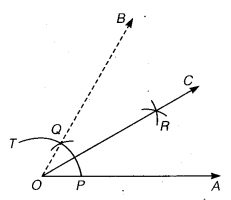1. Draw a ray OA.
2. With centre O any radius draw an arc PT with the help of compasses, cutting ray OA at P.
3. With centre P and the same radius draw an arc cutting the arc PT at Q.
4. Join OQ and produce it to obtain ray OB.
Then, ∠AOB = 60°.
5. With centre P and radius >$\frac { 1 }{ 2 }$ PQ, draw an arc in the interior of ∠AOB.
6. With centre Q and the same radius, as in step 5, draw another arc intersecting the arc in step 5 at R.
7. Join OR and produce it on any point C.
Then, ∠AOC = 30°

(c) Step of Construction: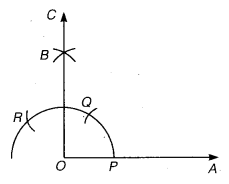1. Draw a ray OA.
2. With O as centre and any convenient radius, draw an arc cutting at P.
3. With P as centre and same radius, draw an arc cutting the arc of step 2 at Q.
4. With Q as centre and the same radius as in steps 2 and 3, draw an arc cutting the arc drawn in step 3 at R.
5. With Q as centre and the same radius, draw an arc.
6. With R as centre and the same radius, draw an arc cutting the arc drawn in step 5 at B.
7. Join O to B and produce it to any point C.
Then, ∠AOC =90°

(d) Steps of Construction: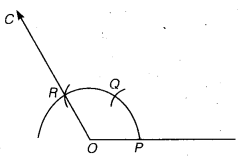1. Draw a ray OA.
2. With O as centre and any convenient radius, draw an arc cutting OA at P.
3. With P as centre and the same radius draw an arc cutting the first arc at Q.
4. With Q as centre and the same radius, draw an cutting the arc drawn in step 2 at R.
5. Join AR and produce it to any point C.
Then, ∠AOC so obtained is of 120°.

(e) Steps of Construction:

1. Draw ∠AOC = 90° by following the steps given in part (iii) above,
2. Draw OE, the bisector of ∠AOC.Then, ∠AOD so obtained is the required angle of 45°.

(f) Steps of Construction: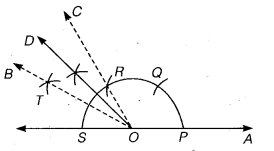1. Draw ∠AOC = 120°
∠AOB =150°.
2. Draw OD, bisector of ∠COB.
Then,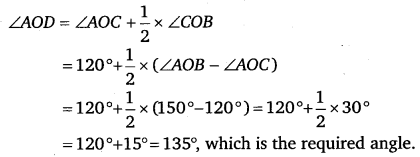Or

Steps of Construction: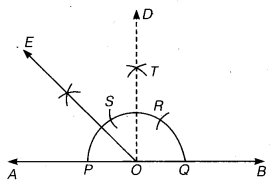1. Draw a line AB and mark a point O on it.
2. With centre O and any convenient radius draw a semi-circle cutting OA and OB at P and Q respectively.
3. With Q as centre and same radius, draw an arc cutting the semi-circle as R.
4. With R as centre and same radius, draw an arc cutting the semi-circle of step 2 at S.
5. With R as centre and same radius draw an arc.
6. With S as centre and same radius draw an arc cutting the arc drawn in step 5 at T. Join OT and produce it to D such that ∠BOD – ∠AOD = 90°.
7. Draw OE, the bisector of ∠AOD.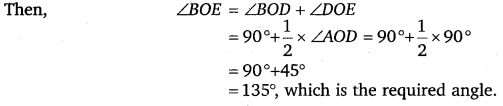Question 6.
Draw an angle of measure 45° and bisect it.

Solution:
Steps of Construction:

1. Draw ∠AOB = 90° by the steps given in question 5 (c).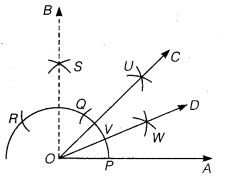2. Draw OC, the bisector of ∠AOB. Then, ∠AOC = 45°.
3. Draw OD, the bisector of ∠AOC. Then, ∠AOD = ∠DOC.

Question 7.
Draw an angle of measure 135° and bisect it.

Solution:
Steps of Construction:

1. Draw ∠EOB = 135° by the steps given in question 5 (f).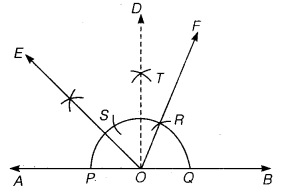2.  Draw OF, the bisector of ∠EOB.
Then, ∠BOF = ∠FOE.

Question 8.
Draw an angle of 70°. Make a copy of it using only a straight edge and compasses.

Solution:
Steps of Construction:

1. Draw an angle 70° with protractor, i. e. ∠POQ = 70°
2. Draw a ray$\overline { AB }$
3. Place the compasses at O and draw an arc to cut the ray of ∠POQ at I and M.
4. Use the same compasses, setting to draw an arc with A as centre, cutting AB at X.
5. Set your compasses setting to the length LM with the same radius.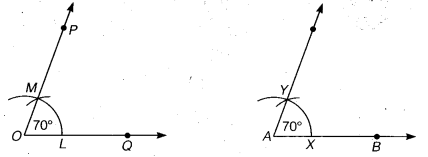6.  Place the compasses pointer at X and draw the arc to cut the arc drawn earlier at Y.
7. Join A7.
Thus, ∠YAX =70°

Question 9.
Draw an angle of 40° copy its supplementary angle.

Solution:
Steps of construction:

1. Draw an angle of 40° with the help of protractor, naming ∠ AOB.
2. Draw a line PQ.3. Take any point M on PQ.
4. Place the compasses at O and draw an arc to cut the rays of ∠ AOB at L and N.
5. Use the same compasses setting to draw an arc O as centre, cutting MQ at X.
6.  Place the compasses at X and draw the arc to cut the arc drawn earlier Y.
7. Join MY.
Thus, ∠QMY = 40° and ∠PMY is supplementary of it.